# proofs .pdf

### File information

This PDF 1.5 document has been generated by TeX / pdfTeX-1.40.18, and has been sent on pdf-archive.com on 25/09/2017 at 21:07, from IP address 75.164.x.x. The current document download page has been viewed 441 times.
File size: 202.5 KB (7 pages).
Privacy: public file

### Document preview

Notes on following Structural Proof Theory by Negri &amp; von Plato
in Agda
Katie Casamento
September 5 2017

Summary
The propositions of intuitionistic propositional logic have a well-known interpretation as types in the simplytyped lambda calculus, under which the rules of a natural deduction proof calculus correspond to lambda term
formers and the cut rule corresponds to capture-avoiding substitution. A similar correspondence between
the rules of sequent calculus and lambda terms, invoking parallel capture-avoiding substitution, suggests a
constructive isomorphism between derivations in the two proof calculi, extending results about either system
to both.
The calculi and results are formalized in Agda, following the informal outlines in Structural Proof Theory
by Negri &amp; von Plato but with ordered lists as sequent contexts instead of multisets; the natural deduction
system still admits the structural rules (weakening, contraction, exchange, and cut) with no other modification, but the formalized sequent calculus includes an exchange axiom justified by an inductive definition
of list equivalence up to permutation and so requires some modifications to the outlined proofs of admissibility. The rules of the proof systems are defined as specializations of a general type of single-succedent
sequent-style derivations, which unifies the Agda-level syntax for building proof trees and simplifies some of
the proofs about the height of derivations. An operational interpreter from closed derivations to Agda terms
is given for each system.

1

Agda Framework

The Agda code is built on top of a general type of derivations parameterized over a type of logical rules.
Formulas are defined as an algebraic data type parameterized over a type of atoms (Formula : Set → Set),
and a sequent is a pair containing an ordered list of premiss formulas and a succedent formula (Sequentα =
ListFormulaα × Formulaα ). It’s useful in the definition of G3ip to be able to define exchange as a rule
that adds no height to a proof tree, so the set of rules of a calculus is modeled as a three-place relation
A : ListSequentα → N → Sequentα → Set, where a value of type Γ An (∆ ` A) is a rule relating premiss
sequents Γ to conclusion ∆ ` A that adds height n to a derivation when it’s applied. Derivations ( ⇒nR ,
or ⇒R unsized) are then defined inductively as constructed by a rule and a list of derivations of the
premisses of the rule.
⇒nR : ListFormulaα → Formulaα → Set
^


# : Γ An (∆ ` A) →
Θ ⇒nR X → ∆ ⇒m+n
A
R
(Θ`X)∈Γ

The height of a derivation can be tracked as a type-level index, but there’s a tradeoff involved: some
admissibility proofs (like contraction in G3ip) are much more straightforward when the height of a derivation
can be used as a terminating argument in structural induction, but some other admissibility proofs (like cut
in G3ip) go through more simply without the burden of formalizing the effect the transformation has on the
height of derivations. I used two different types and wrote functions to convert between them. The height
index of a sized derivation is an upper bound, since an empty list of premiss derivations at the leaves can be

1

taken as containing derivations of any height; one nice consequence of this definition of a derivation is that
n
the function raise : m ≤ n → Γ ⇒m
R A → Γ ⇒R A to witness this property can be defined generically over
derivations in any arbitrary logic.
Negri &amp; von Plato use multisets for sequent contexts, obviating the structural rule of exchange in their
calculi, but with a type of multisets in Agda almost every usually-definitional multiset equality has to
be proven and invoked explicitly, so I went with ordered list contexts instead. In natural deduction, all
the structural rules are derivable with list contexts anyway, so they don’t complicate anything; in G3ip,
I included the exchange rule to compensate. Since applications of the exchange rule show up in the same
places where the multiset equality proofs would be required, the proof burden is about the same, but it keeps
the presentation of everything relatively simple by working within the framework and list contexts turn out
to play nicely with the G3ip structural admissibility proofs.

2

Natural Deduction

Natural deduction systems are characterized by introduction and elimination rules for logical connectives,
which describe how a proof of a proposition involving a connective is created or consumed. The rules of an
intuitionistic natural deduction system can be interpreted as term formers in a lambda calculus extended
with constructors and eliminators for product types and coproduct types and an eliminator for a bottom
type, which gives rise to an isomorphism between the set of valid derivations of any given proposition and
the set of lambda terms of the equivalent type.

2.1

Rules
(v : A) ∈ Γ
Ax
Γ`v:A
Γ ` e1 : A
Γ ` e2 : B
∧I
Γ ` (e1 , e2 ) : A ∧ B

Γ`e:⊥
⊥E
Γ ` void(e) : A

Γ`e:A∧B
∧E L
Γ ` fst(e) : A

Γ`e:A
∨I L
Γ ` left(e) : A ∨ B

Γ`e:A∧B
∧E R
Γ ` snd(e) : B

Γ`B
∨I R
Γ ` right(e) : A ∨ B

v1 : A, Γ ` e1 : C
v2 : B, Γ ` e2 : C
Γ ` e3 : A ∨ B
∨E
Γ ` case(v1 .e1 , v2 .e2 , e3 ) : C
v : A, Γ ` e : B
⊃I
Γ ` λv.e : A ⊃ B

Γ ` e1 : A ⊃ B
Γ ` e2 : A
⊃E
Γ ` e1 e2 : B

v, v1 , and v2 in the ∨E and ⊂ I rules are the names that are bound in the corresponding expressions
by the construction. In the Agda implementation, bindings are nameless and the judgment (v : A) ∈ Γ is
represented by a type of proofs of list inclusion, which behave computationally as de Brujin indices.

2.2

The relation Γ ⊆ ∆ = ∀A. A ∈ Γ → A ∈ ∆ works as a general form of embedding that encompasses
weakening, contraction, and exchange; the only nontrivial cases in embed : Γ ⊆ ∆ → Γ ⇒N A → ∆ ⇒N A
are ⊃ I and ∨E, where a lemma Γ ⊆ ∆ → v : A, Γ ⊆ v : A, ∆ is used to push the embedding under the
binders. The same pattern of proof applies to the cut rule, which is generalized to parallel substitution of the
form Γ b ∆ = ∀A. A ∈ Γ → ∆ ⇒N A and an associated function subst : Γ b ∆ → Γ ⇒N A → ∆ ⇒N A.

2.3

Evaluation

Given a mapping τ from atomic propositions to types, [ ]τ is an inductively-defined Agda type taking general
propositions to types, describing a model of intuitionistic propositional logic in Agda.

2

[P ]τ = τ P
[⊥]τ = Void
[A ∧ B]τ = [A]τ × [B]τ
[A ∨ B]τ = [A]τ ] [B]τ
[A ⊃ B]τ = [A]τ → [B]τ
A total and type-preserving big-step evaluator taking derivations to Agda terms, showing natural deduction consistent with respect to this fragment of Agda, follows from the interpretation of derivations as
lambda terms.
^
eval :
[X]τ → (Γ ⇒N A) → [A]τ
X∈Γ

eval
eval
eval
eval
eval
eval
eval
eval
eval
eval

3

ρ
ρ
ρ
ρ
ρ
ρ
ρ
ρ
ρ
ρ

(Axe
(∧I
(∨I L
(∨I R
(⊃ I
(∧E L
(∧E R
(∨E
(⊃ E
(⊥E

#
#
#
#
#
#
#
#
#
#

[])
[e1 , e2 ])
[e])
[e])
[e])
[e])
[e])
[e1 , e2 , e3 ])
[e1 , e2 ])
[e])

=
=
=
=
=
=
=
=
=
=

index ρ e
(eval ρ e1 , eval ρ e2 )
inj1 (eval ρ e)
inj2 (eval ρ e)
λx. eval (x :: ρ) e
proj1 (eval ρ e)
proj2 (eval ρ e)
either (λx. eval (x :: ρ) e1 ) (λx. eval (x :: ρ) e2 ) (eval ρ e3 )
eval ρ e1 (eval ρ e2 )
void (eval ρ e)

Sequent Calculus (G3ip)

Sequent calculi are characterized by left and right rules, which describe how connectives are introduced on the
left side of a sequent (in the context of assumptions) or on the right side (in the formula being derived). The
only rule that moves a proposition from the left to the right is the axiom rule, which is restricted to atomic
propositions, so all derivations are constructed by decomposing assumptions into their constituent atoms
and then building up conclusions from the atoms. That regularity gives rise to the subformula principle,
which can be used as a termination measure for proof search procedures and consistency proofs that are not
obviously terminating in natural deduction.
Negri &amp; von Plato give a calculus called G3ip, with multiset contexts; the Agda code uses the same
definition but with list contexts and extended with an exchange rule.

3.1

Rules
Γ ⊃⊂ ∆
Γ`A
Exch
∆`A
A, B, Γ ` C
∧L
A ∧ B, Γ ` C
A, Γ ` C
B, Γ ` C
∨L
A ∨ B, Γ ` C

P, Γ ` P

Ax

⊥, Γ ` A

⊥L

Γ`A
Γ`B
∧R
Γ`A∧B
Γ`A
L
Γ ` A ∨ B ∨R

A ⊃ B, Γ ` A
B, Γ ` C
⊃L
A ⊃ B, Γ ` C

Γ`B
R
Γ ` A ∨ B ∨R

A, Γ ` B
⊃R
Γ`A⊃B

The Ax rule is restricted to acting on the head of the context, since the more general rule that targets any
assumed atom is recoverable from this rule and the exchange rule. The judgment Γ ⊃⊂ ∆ is represented in
Agda as an inductive definition of list equivalence up to permutation (from Twar van Laarhoven), in terms
of a type A I Γ ≈ ∆ witnessing that there exists some index where A can be inserted into Γ to get ∆.
3

A I Γ ≈ A, Γ

Here

 ⊃⊂  N il

3.2

AIΓ≈∆
T here
A I B, Γ ≈ B, ∆

A I ∆ ≈ ∆0
Γ ⊃⊂ ∆
Cons
A, Γ ⊃⊂ ∆0

The Agda proofs of admissibility for weakening, contraction, and cut closely follow Negri &amp; von Plato. The
literal translation of contraction (A, A, Γ ⇒G B → A, Γ ⇒G B) and cut (Γ ⇒G A → A, ∆ ⇒G B →
Γ, ∆ ⇒G B) into a setting with ordered list contexts only cover the case where the relevant formula is at
the head of the context, which isn’t enough in the presence of the exchange rule, so they’re defined in terms
of a computational definition of removal of a single index from a list: contraction is A ∈ Γ → A ∈ Γ − A →
Γ ⇒G B → Γ − A ⇒G B, and cut is Γ ⇒G A → A ∈ ∆ → ∆ ⇒G B → Γ, ∆ − A ⇒G B. Since the principal
formula, if there is one, is always at the head of the context, the division of the admissibility proofs into cases
is straightforward pattern matching, with the caveat that the contraction proof needs to check in separate
cases whether either instance of A in the context is principal.
Several lemmas about permutations come into play. In particular, the reason the more general embedding
rule doesn’t work in G3ip is because the exchange case in the contraction and cut admissibility proofs needs
the relation in question to be symmetric and closed under removal of the same element from both sides. A
conversion from A ∈ Γ to A I Γ − A ≈ Γ bridges the gap between the index arguments to contract/cut
and the permutation lemmas.
Negri &amp; von Plato note that the contraction transformation is easier to show terminating when it’s
defined as preserving the height of the input derivation, and this carries over to the Agda implementation:
the principal formula cases for ∨L and ⊃ L recurse over the results of ∨LInv /⊃ LInv applied to strict
subderivations, which preserves the decreasing height of the subderivation but not the syntactic property
of being a subderivation. The cut proof is conveniently structurally recursive without a height metric, with
the derivation serving as the decreasing argument in every recursive call except for the lowermost cut in the
⊃ R case, which decreases the head formula A ⊃ B to A.
The syntactic consistency and disjunction properties from Negri &amp; von Plato also carry over as straightforward pattern matching; the left rules are ruled out in an empty context because there has to be a head of
the context for the principal formula to be at, and the exchange rule is done away with by the observation
that the only permutation on an empty context is the identity permutation. The definition of a Harrop
formula is represented as an inductive predicate over the formula type, and the disjunction property for a
context of Harrop formulas is defined as a recursive search for the ∨R instance implied by the conclusion of
the derivation, noting that the otherwise problematic ∨L case is ruled out by the Harrop predicate on the

3.3

Evaluation

A big-step evaluator can be defined for G3ip in the same style as for natural deduction, using the same
interpretation of formulas as types. The right rules for each connective are identical to the introduction rules
in natural deduction, and the Ax rule is the natural deduction axiom rule restricted to an atomic formula at
the head of the context, so those rules can all be evaluated the same as in natural deduction. The right rules
can be understood as recursively evaluating subterms in an unchanged environment and doing something
with the resulting values; dually, the left rules do something to the environment and then tail-recurse over
subterms. Pattern matching on an application of a left rule splits the context into a principal (head) formula
and a tail, revealing a value at the head of the evaluation environment typed in accordance with the principal
formula.
eval
eval
eval
eval
eval

((x1 , x2 ) :: ρ)
(inj1 x :: ρ)
(inj2 x :: ρ)
(f :: ρ)
(() :: ρ)

(∧L
(∨L
(∨L
(⊃ L
(⊥L

#
#
#
#
#

[e])
[e1 , e2 ])
[e1 , e2 ])
[e1 , e2 ])
[])

4

= eval
= eval
= eval
= eval

(x1 :: x2 :: ρ) e
(x :: ρ) e1
(x :: ρ) e2
(eval (f :: ρ) e1 :: ρ) e2

The syntax () in place of an argument says in Agda that there are no possible constructors for the type of
the argument, so the pattern is impossible and there’s no need to provide a right-hand side to the definition.

3.4

Interpretation in STLC

Since the sublanguage of Agda described by [ ] is effectively an embedding of STLC, the rules of the evaluator
suggest an interpretation of sequent calculus derivations as lambda terms. The evaluator reads as a bottomup account of the action of the G3ip rules, pattern matching over terms and recursing into subterms; the
rules read as a top-down process of composing subterms into terms.
Ax and the right rules can all be given the same interpretations as the corresponding natural deduction
rules (with Ax appropriately restricted). ∨L and ⊥L are identifiable as ∨E and ⊥E restricted to case only
over terms represented by bound variables, so their interpretations are as restricted forms of the interpretations of their respective elimination rules. The other left rules (∧L and ⊃ L) act somewhat differently
from the corresponding elimination rules: they act on the context of a well-typed term, which can be seen
as inducing a structural transformation over the term to adapt it to a different context. Under this interpretation, ∧L is a parallel substitution and ⊃ L is a substitution composed with an application, and both
are restricted to acting on variables like ∨E and ⊥E are.
v1 : A, v2 : B, Γ ` e : C
∧L
v : A ∧ B, Γ ` e[v1 /fst(v), v2 /snd(v)] : C

v1 : A ⊃ B, Γ ` e1 : A
v2 : B, Γ ` e2 : C
⊃L
v : A ⊃ B, Γ ` e2 [v2 /(v e1 [v1 /v])] : C

The A ⊃ B assumption in the left premiss of ⊃ L becomes a bound variable containing the function
that will be applied to that term, which is to say that in an application e1 e2 the term e2 can contain
references to e1 . The view of ⊃ L as a form of composition is clearer in the version without the repeated
A ⊃ B assumption in the left premiss, which can be seen as (β-equivalent to) applying the composition of
two functions to an argument.
Γ ` e1 : A
v2 : B, Γ ` e2 : C
⊃L
v : A ⊃ B, Γ ` ((λv2 .e2 ) ◦ v) e1 : C
The term syntax for ∧L, ∨L, and ⊥L can be unified with a general form for pattern matching over
variables, but ⊂ L mysteriously resists this characterization.
v1 : A, v2 : B, Γ ` e : C
∧L
v : A ∧ B, Γ ` case v of {(v1 , v2 ) → e} : C
v1 : A, Γ ` e1 : C
v2 : B, Γ ` e2 : C
∨L
v : A ∨ B, Γ ` case v of {left v1 → e1 ; right v2 → e2 } : C
v : ⊥, Γ ` case v of {} : A

4
4.1

⊥L

Conversion between calculi
G3ip to natural deduction

Natural deduction derivations correspond to lambda terms without explicit substitution, which admit a
substitution operator, and sequent calculus derivations can be interpreted as lambda terms constructed
with certain forms of explicit substitution; this suggests a conversion from sequent calculus to natural
deduction by way of the derived substitution rule. ∧L is translated by the parallel substitution operator,
Ax
with a substitution A, B, Γ b A ∧ B, Γ that takes the A assumption to A ∧ B, Γ ` A ∧ B ∧E L and the B
A ∧ B, Γ ` A
Ax
A

B,
Γ
`
A

B
assumption to
∧E R .
A ∧ B, Γ ` B

5

A, B, Γ ` C
∧L
A ∧ B, Γ ` C

..
.
A, B, Γ c A ∧ B, Γ
A, B, Γ ` C
Subst
A ∧ B, Γ ` D
⊃ L invokes ⊃ E and cut (with the context shared between premisses), and ∨L and ⊥L are direct
applications of ∨E and ⊥E.
A ⊃ B, Γ ` A
B, Γ ` C
⊃L
A ⊃ B, Γ ` C

Ax
A ⊃ B, Γ ⊃ A ⊃ B
A ⊃ B, Γ ` A
⊃E
A ⊃ B, Γ ` B
A ⊃ B, Γ ` C

B, Γ ` C
Embed
B, A ⊃ B, Γ ` C
Cut

A, Γ ` C
B, Γ ` C
∨L
A ∨ B, Γ ` C

B, Γ ` C
Embed
B, A ∨ B, Γ ` C
A ∨ B, Γ ` C

A, Γ ` C
Embed
A, A ∨ B, Γ ` C

⊥, Γ ` A

A ∨ B, Γ ` A ∨ B

Ax
∨E

⊥L

Ax
⊥, Γ ` ⊥
⊥E
⊥, Γ ` A

4.2

Natural deduction to G3ip

The natural deduction definition doesn’t include any structural axioms, so there’s no exchange case to
handle in this direction. The assumption case invokes the lemma from Negri &amp; von Plato that derives any
assumption in a context. ∨E is ∨L followed by a substitution. ⊥E is shown admissible by noting that no
right rule can conclude ⊥ so the derivation must consist only of left rules and Ax with an instance of Ax
deriving ⊥ at one of the leaves; the inverses of ∧R and ⊃ R are derived similarly, except no Ax instance
can derive a non-atomic formula, so the induction stops at an instance of ∧R/⊃ R that has the relevant
assumption.
Γ`A∧B
∧E L
Γ`A

Γ`A∧B
∧E R
Γ`B

Γ ` A ∧ B ∧R L
Inv
Γ`A

Γ ` A ∧ B ∧R R
Inv
Γ`B
6

A, Γ ` C

B, Γ ` C
Γ`C

Γ`A∨B

∨E

Γ`A∨B

A, Γ ` C
B, Γ ` C
∨L
A ∨ B, Γ ` D
Cut
Γ`C

Γ`A⊃B
Γ`A
⊃E
Γ`A

Γ`A

4.3

Γ`A⊃B
⊃ RInv
A, Γ ` B
Cut
Γ`A

Consequences

The embedding and parallel substitution rules from natural deduction are immediately admissible in G3ip.
This is a significantly simpler proof than the the native admissibility proofs for these rules in G3ip, which
would have to derive instances of the structural rules by observation of the finite Agda functions witnessing
the relations. The consistency and disjunction results are already proven by the evaluator for natural
deduction, but the translation also provides an alternate proof by way of G3ip.

5

Conclusion and further work

The experience of working on these proofs in Agda went overall pretty smoothly. I had a few false starts
in handling the change from multisets to lists in G3ip, initially assuming I’d be able to use some variation
on the embedding rule from natural deduction as an axiom, but they were worthwhile detours that revealed
which properties are necessary in the relation backing the exchange rule. The evaluator for G3ip made
surprisingly clear the duality of the left and right rules in their respective roles of breaking down assumptions
and constructing conclusions, and I feel like I have a much better understanding of the calculus and the
admissibility proofs from Negri &amp; von Plato for having worked through them case by case.
Working with two different types to accommodate height-preserving transformations incurred a manageable but disappointing syntactic overhead, but I don’t know how to unify them in a definition that
would allow height-preserving functions to be applied to unsized derivations without any conversion; Conor
McBride’s work on ornaments seems like it might be relevant. Other than that, it would be interesting to
try to extend the derivation type to handle multi-succedent sequents to formalize classical logic, and orthogonally formulas and sequents with a notion of bound variables and substitution to formalize predicate logic.
It should also be possible to give a constructive proof that the type of derivations in G3ip of any formula is
finite, inducing a complete search procedure for an inhabitant of any type in STLC.

7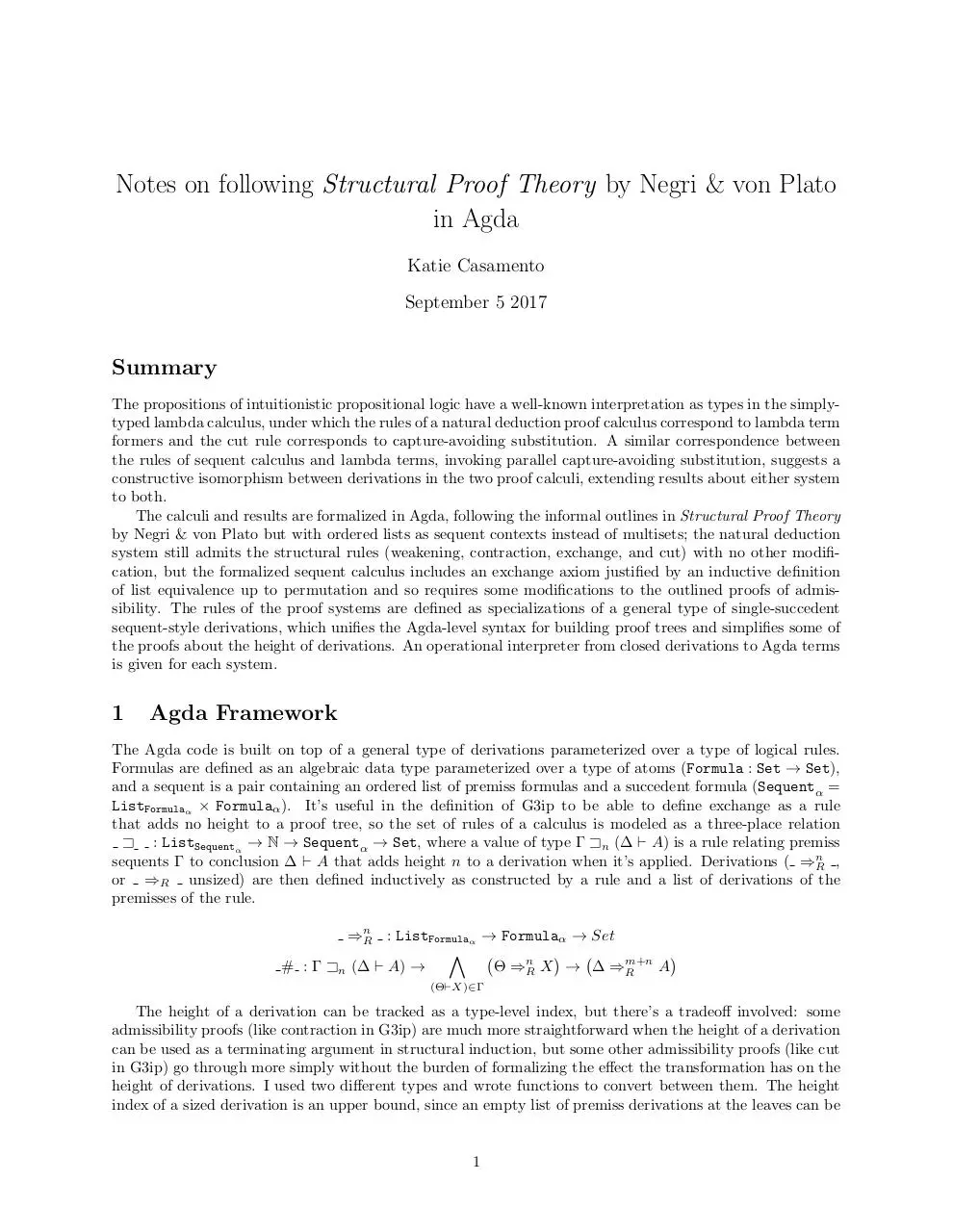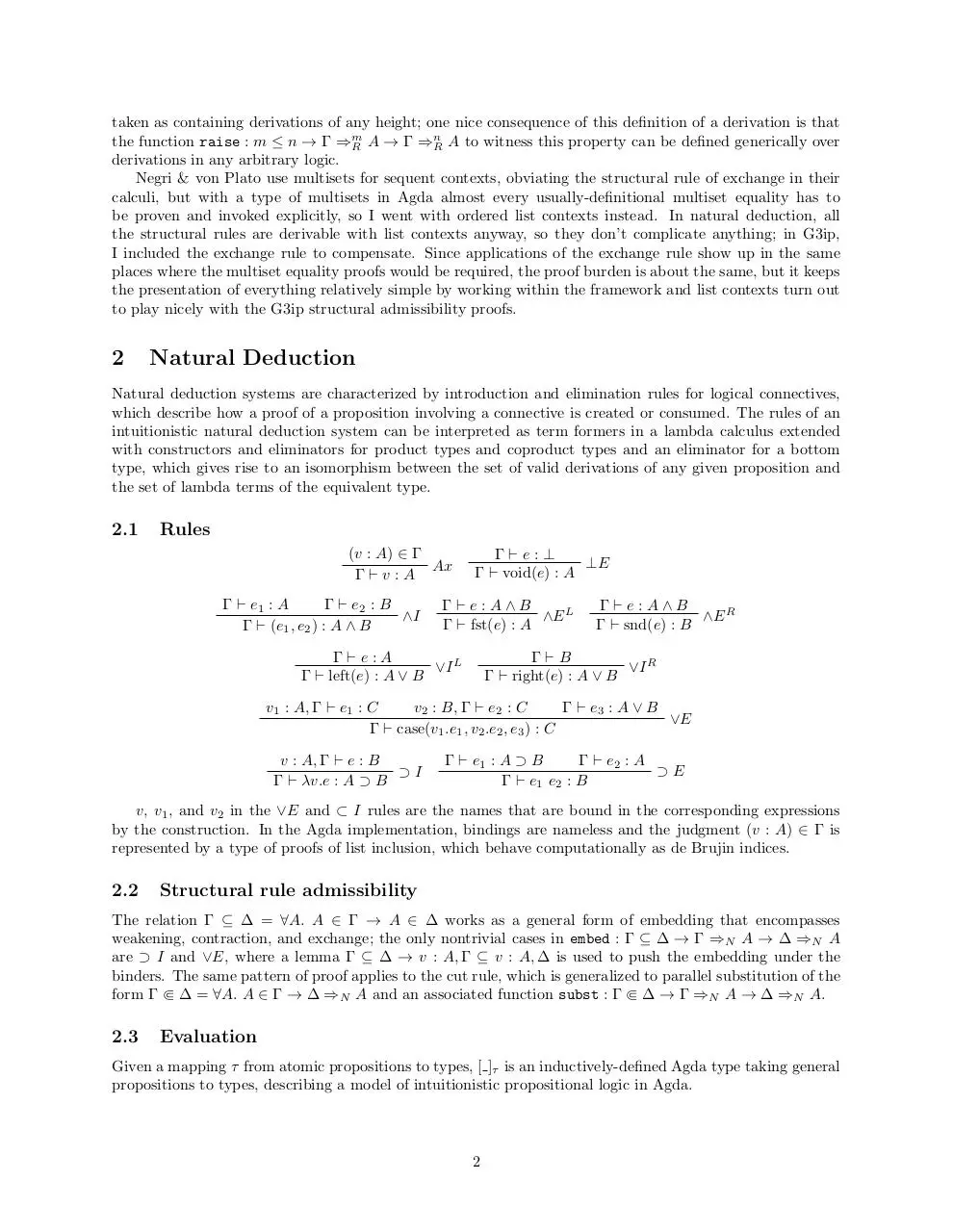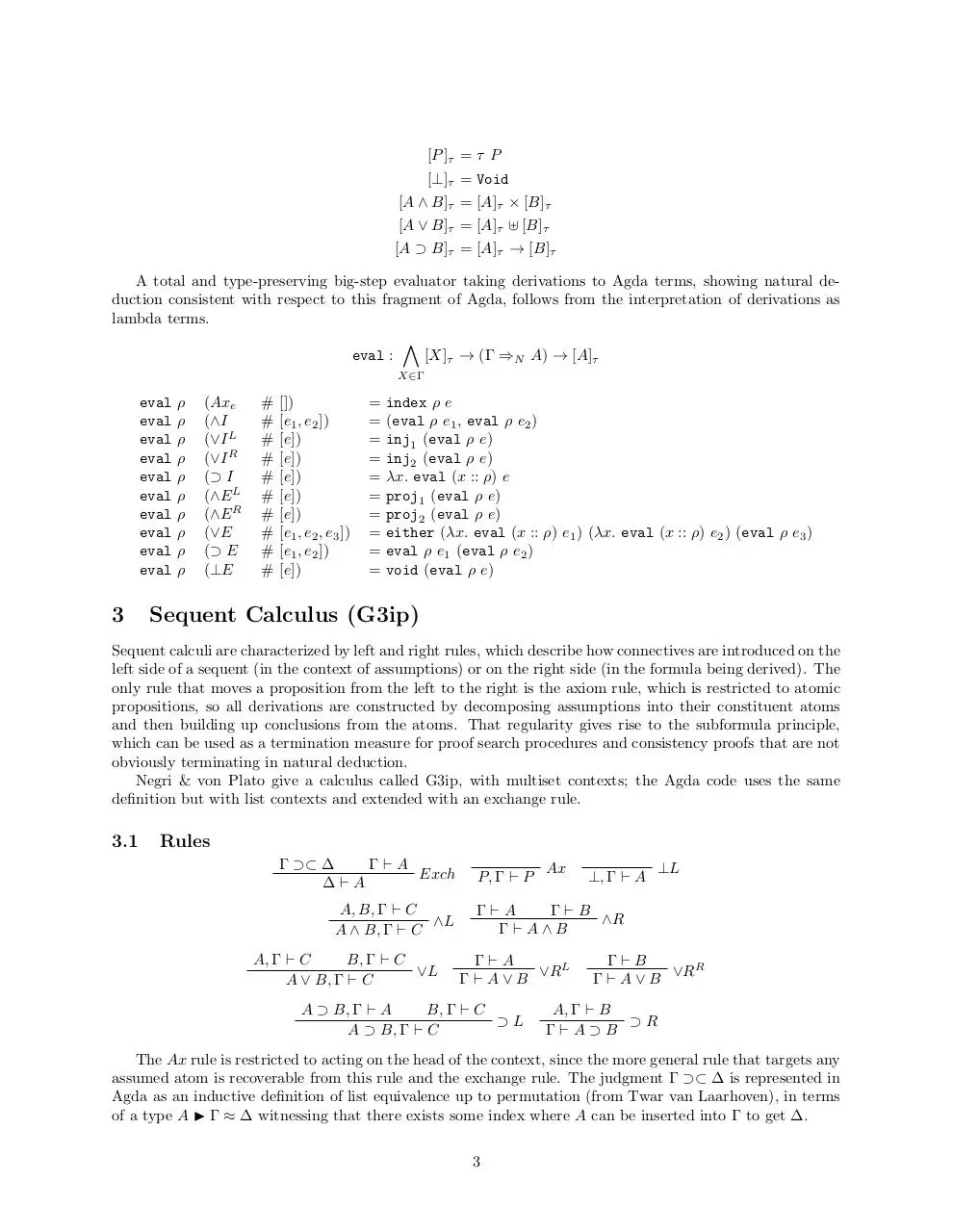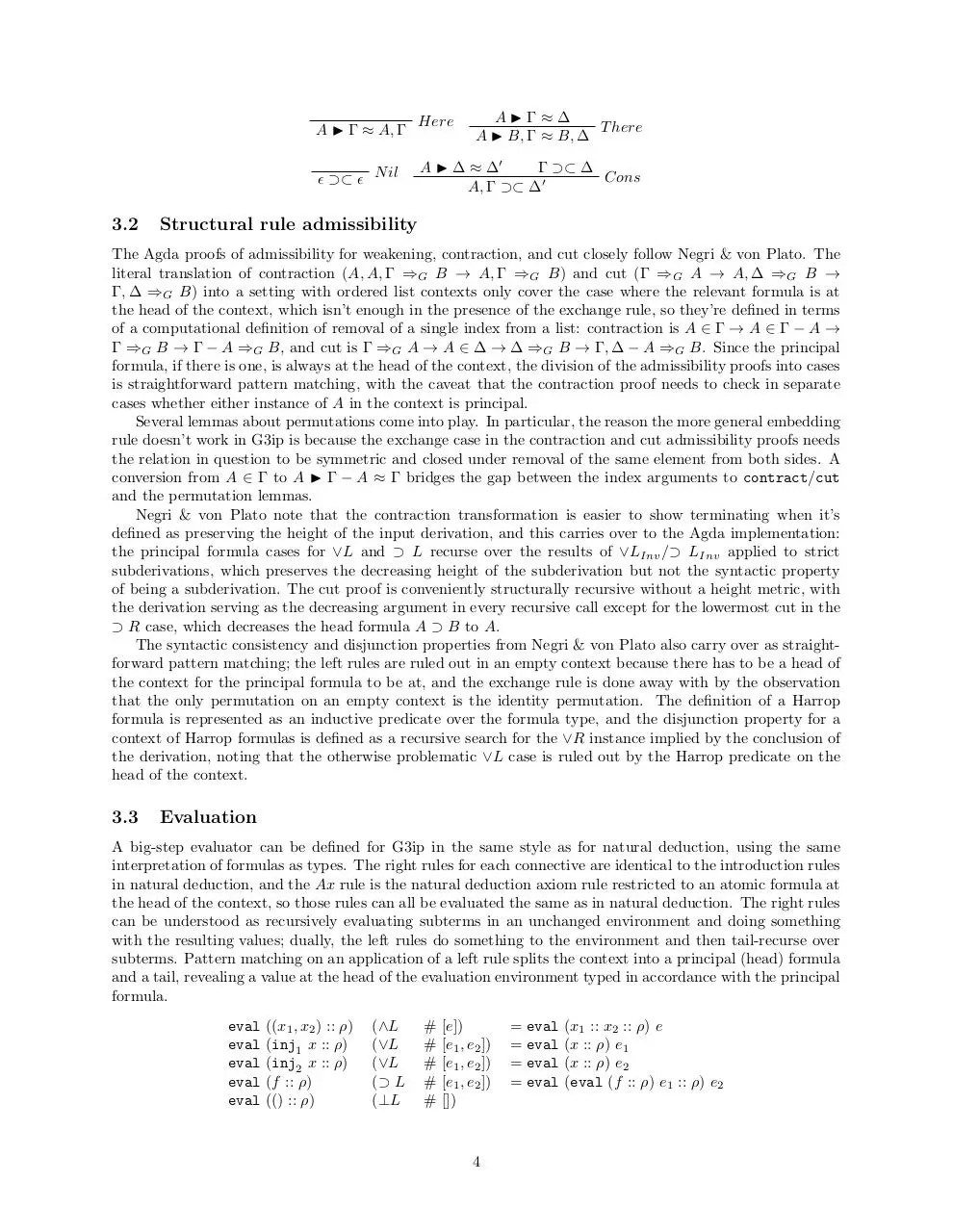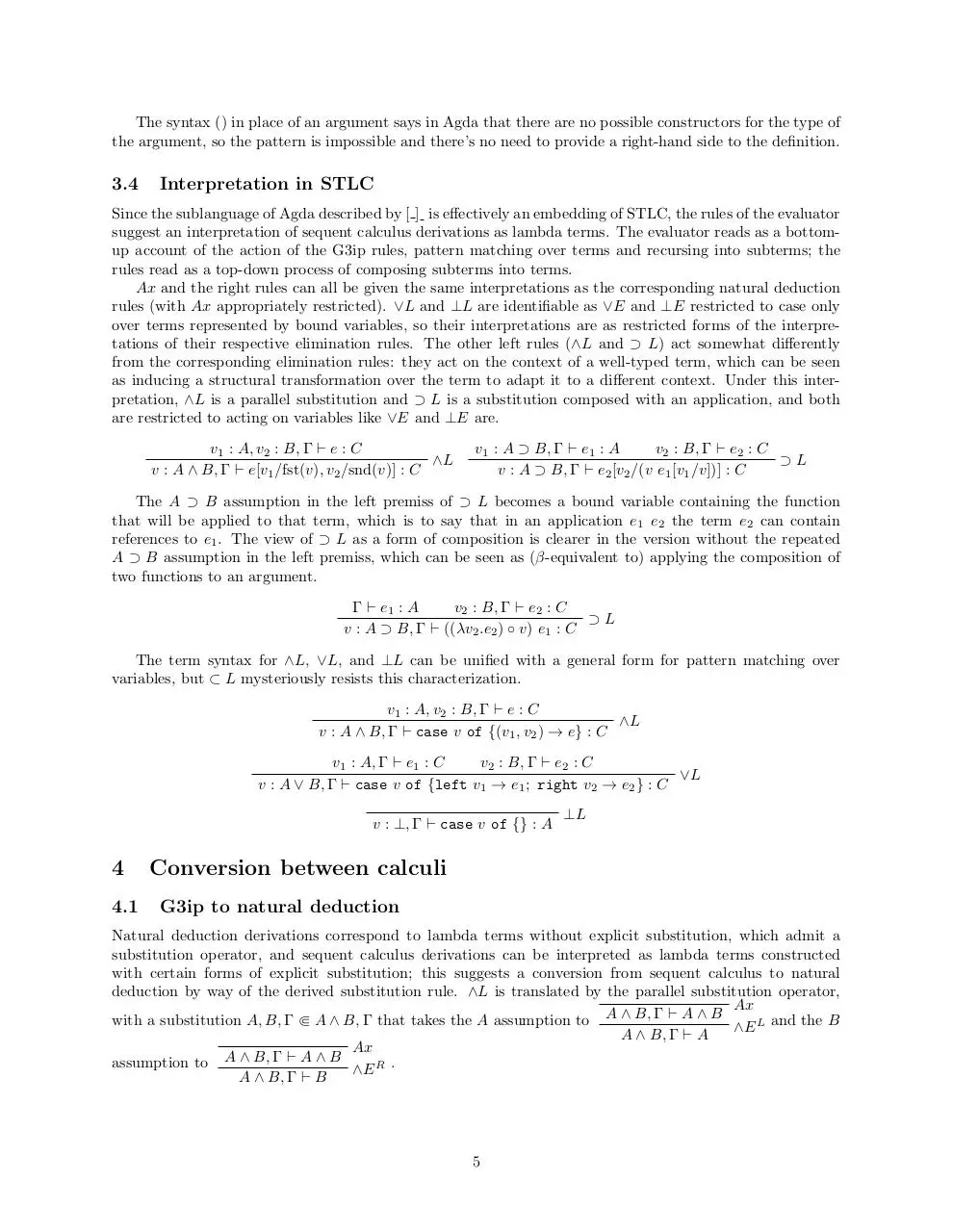proofs.pdf (PDF, 202.5 KB)

### Share on social networks

#### HTML Code

Copy the following HTML code to share your document on a Website or Blog